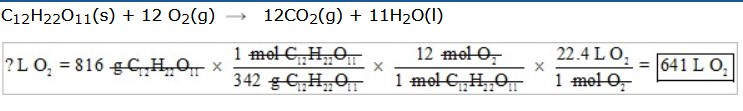# Gas Laws Test

1.  When Joseph Kittinger rode a balloon to a height 18.5 miles in the sky, he ran into trouble. At a height of 43,000 feet the pressure glove on his right hand failed to work. EXPLAIN what happened to his hand based on your knowledge of atmospheric pressure and the gas laws.

2.  Use the kinetic theory to explain why a helium filled balloon expands when it is brought inside to a warm room from the cold outdoors.

3. Define “ideal gas.”

4. When atmospheric pressure increases, how does the height of the column of mercury change?

5. If A and B are directly proportional and the value of A becomes 1/3 as much, what happens to the value of B?

7. Tire manufacturers recommend checking air pressure when tires are cold, before driving. Why?

8. At STP, 22.4 L of N2 contain how many molecules?

9. Ammonia (NH3) and sulfur dioxide (SO2) are both gases with readily distinguishable odors. If a cylinder of each were opened at the same time in a draftless room, which odor would you expect to smell first? Explain.

10. Calculate the pressure exerted by a 120 pound woman wearing high heels with a surface area of 0.02 square inches as she walks across a wooden floor.

11. What volume does 2.50 moles of carbon monoxide occupy at 50.5 kPa pressure and 20.0 °C?

12. At 800 mm Hg, a gas has a volume of 380 L. What is its volume at standard pressure?

13. A quantity of gas has a volume of 121 L when confined under a pressure of 2.50 atm at a temperature of 20.0 °C. At what pressure will its volume be 30.0 L at 25.0 °C?

14. At constant pressure, the volume of a gas is increased from 150.0 L to 300.0 L by heating it. If the original temperature of the gas was 20.0 °C, what will its final temperature (in Kelvins) be?

15. A quantity of gas is collected over water at 15 °C. The manometer indicated a pressure of 24.0 kPa. What would be the pressure of the dry gas?

16. How many liters of pure oxygen at STP are consumed by a human being in 24 hours if the human body requires daily energy that comes from metabolizing 816 grams of sucrose (C12H22O11)?

1. Because of decreased atmospheric pressure, his hand swelled.
2. Increasing the temperature of a gas means that the particles have more kinetic energy. Consequently, the collisions of the gas molecules with the walls of the container occur more often and are more forceful, so the gas pressure would increase. Because it is assumed that the pressure inside the warm room and outside is the same, the volume must increase to reduce the pressure to its original value.
3. An ideal gas is imaginary, and its behavior conforms exactly to the assumptions of the kinetic theory.
4. increases
5. B becomes 1/3 as much.
6. Avogadro’s law states that equal volumes of different gases at the same temperature and pressure contain the same number of molecules.
7. As you drive, the temperature of the air inside the tire increases, increasing the pressure since the volume remains constant.
8. 6.02 x 1023
9. Because both gases are at the same temperature, they each possess the same average kinetic energy.  Since KE = 1/2 mv², the more massive gas will have the smaller velocity.  The molar mass of ammonia is 17.0 g/mol and the molar mass of sulfur dioxide is 64.0 g/mol. Therefore, the ammonia will be smelled first since it is the less massive of the two gases.
10. Pressure = force/area = 120 pounds/0.02 in² = 6000 pounds/in² (psi)
11.12. V2 = (P1 x V1) / P2
V2 = (800 mm Hg x 380L)/760 mm Hg = 400 L
13. P2 = (2.50 atm x 121 L x 298 K)/(293 K x 30.0 L) = 10.3 atm
14. T2 = (V2T1)/V1 = (300.0 L x 293 K)/150 L = 586 K
15. 24.0 kPa – 1.7 kPa = 22.3 kPa
16.source